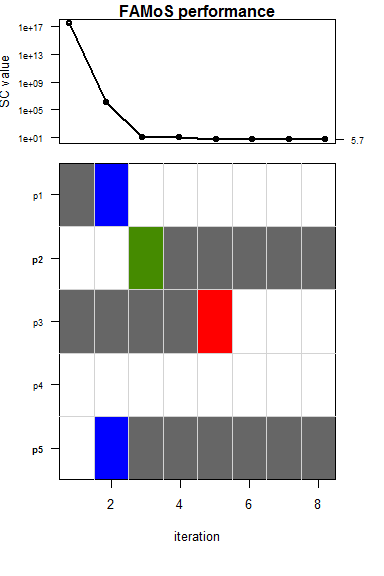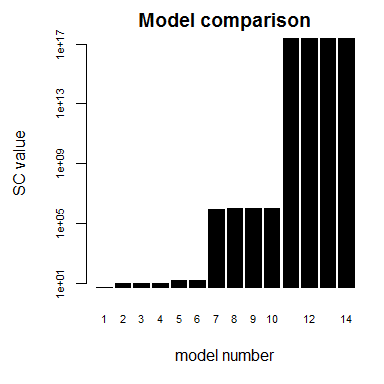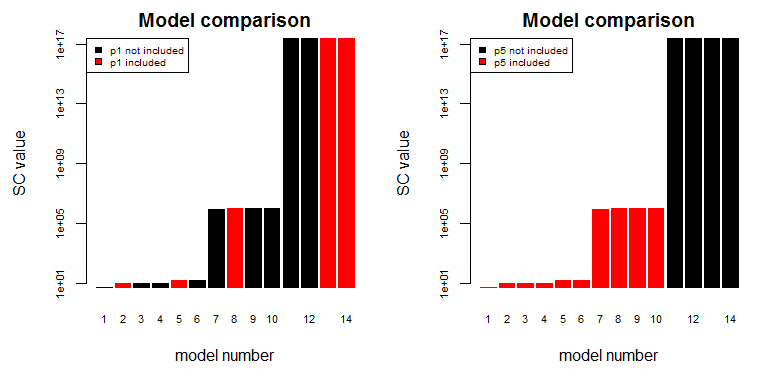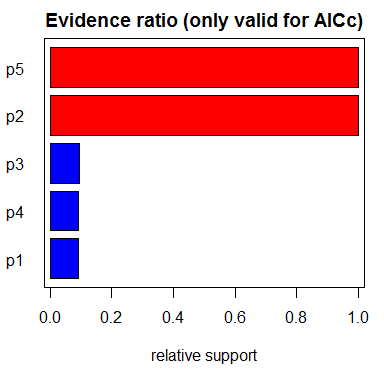# Plotting the results of the model selection process

The FAMoS package comes with a variety of functions that allow to plot the outcome of the model selection algorithm in a convenient way. These functions can be helpful to assess the performance of the model selection algorithm and help in interpreting the support of each model parameter.

For this tutorial we will first run our general example again:

``````library(FAMoS)

#setting data
true.p2 <- 3
true.p5 <- 2
sim.data <- cbind.data.frame(range = 1:10,
y = true.p2^2 * (1:10)^2 - exp(true.p5 * (1:10)))

#define initial parameter values and corresponding test function
inits <- c(p1 = 3, p2 = 4, p3 = -2, p4 = 2, p5 = 0)

cost_function <- function(parms, binary, data){
if(max(abs(parms)) > 5){
return(NA)
}
with(as.list(c(parms)), {
res <- p1*4 + p2^2*data\$range^2 + p3*sin(data\$range) + p4*data\$range - exp(p5*data\$range)
diff <- sum((res - data\$y)^2)

#calculate AICC
nr.par <- length(which(binary == 1))
nr.data <- nrow(data)
AICC <- diff + 2*nr.par + 2*nr.par*(nr.par + 1)/(nr.data - nr.par -1)

return(AICC)
})
}

#set swap set
swaps <- list(c("p1", "p5"))

#perform model selection
res <- famos(init.par = inits,
fit.fn = cost_function,
homedir = tempdir(),
method = "swap",
swap.parameters = swaps,
init.model.type = c("p1", "p3"),
optim.runs = 1,
data = sim.data) ``````

To assess the performance of a specific FAMoS run, the function famos.performance can be used. This generates a plot showing the improvement in the information criterion over the course of each FAMoS iteration along with the corresponding best model.

``famos.performance(input = res\$mrun, path = tempdir())``The boxes in the lower plot what happened during each FAMoS iteration. Here, parameter p1 was swapped for p5 in the second iteration (blue box), parameter p3 was added in the third iteration (green box) and parameter p2 was removed in the fifth iteration (red box). After that, FAMoS tried to find better models with the succession of the methods backward, forward and swap, but did not succeed. Therefore the model selection procedure was terminated after the eighth iteration. The best model, therefore consists of the parameters p3 and p5 and the corresponding AICc is shown on the right axis of the upper plot.

Note that the performance of FAMoS can also be checked while the algorithm is still running. After the third iteration, a plot generated by famos.performance will be stored in the “FAMoS-Results/Figures/” folder. This plot will be updated at the end of each iteration. Alternatively, the function can also be called in the console.

In a next step, we can check how the tested models are distributed in terms of the used information criterion (here: AICc). To this end, we call the function sc.order.

`` fig.sc <- sc.order(input = tempdir(), mrun = res\$mrun)``We can also use this function to check how certain parameters affect the value of the information criterion

`````` par(mfrow = c(1,2))
fig.sc1 <- sc.order(input = tempdir(), mrun = res\$mrun, colour.par = "p1")
fig.sc2 <- sc.order(input = tempdir(), mrun = res\$mrun, colour.par = "p5")``````Here, we can see that all models that contain p5 perform much better than models that don’t have this parameter.

We can analyse the support of each parameter even more closely by calculating the Akaike weights with the function aicc.weights, which gives a normalised description of the importance of parameters. Roughly, values close to 1 indicate high and values close to 0 weak to no support. Parameters with intermediate support might indicate a necessity of having a certain feature in the model to describe the data, but this feature might be achieved by different parameters.

``fig.aicc <- aicc.weights(input = tempdir(), mrun = res\$mrun)``